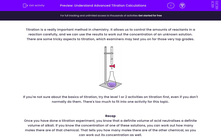### Comprehensive & curriculum aligned

In this worksheet, students will learn how to solve titration problems where there isn't a 1:1 ratio of acid-neutralising alkali, or where the concentrations are given in terms of mass, not moles.Key stage:  KS 4

Year:  GCSE

GCSE Boards:   OCR 21st Century, Eduqas, Pearson Edexcel,

Curriculum topic:   Chemical Analysis, Chemistry of Acids, Chemical Changes

Curriculum subtopic:   How are the Amounts of Chemicals in Solution Measured? Chemistry of Acids Acids

Difficulty level:#### Worksheet Overview

Titration is a really important method in chemistry. It allows us to control the amounts of reactants in a reaction carefully, and we can use the results to work out the concentration of an unknown solution.

There are some tricky aspects to titration, which examiners may test you on for those very top grades.If you're not sure about the basics of titration, try the level 1 or 2 activities on titration first, even if you don't normally do them. There's too much to fit into one activity for this topic.

Recap

Once you have done a titration experiment, you know that a definite volume of acid neutralises a definite volume of alkali. If you know the concentration of one of these solutions, you can work out how many moles there are of that chemical. That tells you how many moles there are of the other chemical, so you can work out its concentration as well.

First difficulty: what if 1 mole of acid doesn't neutralise 1 mole of alkali?

If you neutralise HCl with NaOH, 1 mole of alkali neutralises 1 mole of acid: HCl + NaOH → NaCl + H2O.

The equation for neutralising sulfuric acid with NaOH is H2SO4 + 2NaOH → Na2SO4 + 2H2O. This means that you need two moles of sodium hydroxide to neutralise one mole of acid.

Example: 25.0 cm3 of NaOH (unknown concentration) is neutralised by 10.5 cm3 of H2SO4 (0.1 mols per dm3 concentration). What is the concentration of the sodium hydroxide?

Amount of acid = volume x concentration. Volume = 10.5 but change to litres by multiplying by 1000. Concentration = 0.1.

So, amount of acid = (10.5 ÷ 1000) x 0.1 = 0.001 05 mol.

From the equation, there is twice as much alkali as acid, so amount of alkali = 0.001 05 x 2 = 0.002 10 mol.

The concentration of NaOH = the amount ÷ volume

The concentration of NaOH = 0.002 10 ÷ (25.0 ÷ 1000) = 0.0084 mol per dm3 (also written mol dm-3).

Second difficulty: what if the concentration is in grams per dm3, not moles per dm3?

If the concentration is in grams per dm3, we need to convert grams to moles before doing the titration calculations. We do that using relative formula mass.

Example: 25.0 cm3 of NaOH (unknown concentration) is neutralised by 20.0 cm3 of HCl (7.3 g per dm3 concentration). What is the concentration of the sodium hydroxide in g dm-3?

Work out the number of moles of HCl:

Relative formula mass of HCl = 1 + 35.5 = 36.5. So 1 mol = 36.5 g.

7.3  ÷  36.5 = 0.2, so 7.3 g dm-3 = 0.2 mol dm-3

Total number of moles of HCl = (20.0 ÷ 1000) x 0.2 = 0.004 mol.

Work out the number of moles of NaOH:

Neutralising 1 mol of NaOH needs 1 mol of HCl, so moles of NaOH = 0.004 mol.

Convert the moles of NaOH into grams:

Relative formula mass of NaOH = 23 + 16 + 1 = 40. So 1 mol = 40 g.

So the mass of NaOH = 0.004 x 40 = 0.16 g.

Work out the concentration:

Concentration = mass ÷ volume = 0.16 ÷ (25 ÷ 1000) = 6.4 g dm-3

None of the steps in the calculation are too hard - the difficult thing is doing all of them in the right order to get to the answer.

It's a lot easier to do this if you add words to the number. Writing down what each calculation is trying to do will make it much easier to focus on doing the right things in the right order. It will also help someone reading your work (like an examiner) to understand what you have done.

Let's have a go at some questions now.

### What is EdPlace?

We're your National Curriculum aligned online education content provider helping each child succeed in English, maths and science from year 1 to GCSE. With an EdPlace account you’ll be able to track and measure progress, helping each child achieve their best. We build confidence and attainment by personalising each child’s learning at a level that suits them.

Get started••••